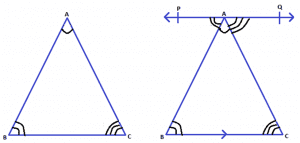# Lines and AnglesQuadrilaterals and Triangles: Angle sum property

A plane figure bounded by finite line segments to form a closed figure is defined as polygon.Based on the number of sides or vertices a polygon can be classified as n-sided polygon. A polygon bounded by three finite line segments is known as triangle. It is the smallest possible polygon. A four-sided polygon is known as a quadrilateral.

The sum of interior angles of any n-sided polygon is given by (n-2) × 180°. Thus, the sum of interior angles of a triangle is 180° and the sum of interior angles of a quadrilateral is 360°. Let us try to prove this mathematically.

Angle Sum Property of Triangles:

Consider a ∆ABC, as shown in the figure below.

Construction: Draw a line

$$\begin{array}{l} \overleftrightarrow {PQ} \end{array}$$
passing through point A such that PQ||BC .Figure 1: Proof of angle sum property of triangles

Since PQ is a straight line, it can be concluded that:

∠PAB + ∠BAC + ∠QAC = 180° ….(1)

Since PQ||BC and AB, AC are transversals to the parallel lines,

Also, ∠QAC = ∠ACB and ∠PAB = ∠CBA (pair of alternate angles)

Substituting the value of ∠QAC and∠PAB in equation (1),

∠ACB + ∠BAC + ∠CBA = 180°

Thus, the sum of interior angles of a triangle is always 180°.

Constructions: Draw diagonal BD as shown below:

Figure 3

From figure 3;

∠B = ∠3 + ∠4 …..(1)
∠D = ∠1 + ∠2 …..(2)

In ∆ABD,

∠A + ∠1 + ∠3 = 180° …………………………(3) (Angle Sum Property of triangles)

Similarly, in ∆BCD,

∠C + ∠2 + ∠4 = 180°………………………….(4) (Angle Sum Property of triangles)